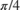# getRBW

Resolution bandwidth of spectrum

## Syntax

RBW = getRBW(estimator)
RBW = getRBW(estimator,Fs)

## Description

example

RBW = getRBW(estimator) returns the resolution bandwidth of the spectral estimate.
RBW = getRBW(estimator,Fs) returns the resolution bandwidth assuming an input sample rate of Fs.

## Examples

collapse all

Compute the power spectrum of a multichannel sinusoidal signal using the dsp.SpectrumEstimator System object™. You can get the vector of frequencies at which the spectrum is estimated using the getFrequencyVector function. To compute the resolution bandwidth of the estimate (RBW), use the getRBW function.

Generate a three-channel sinusoid sampled at 1 kHz. Specify sinusoidal frequencies of 100, 200, and 300 Hz. The second and third channels have their phases offset from the first byand, respectively.

sineSignal = dsp.SineWave('SamplesPerFrame',1000,'SampleRate',1000, ... 'Frequency',[100 200 300],'PhaseOffset',[0 pi/2 pi/4]); 

Estimate and plot the one-sided spectrum of the signal. Use the dsp.SpectrumEstimator object for the computation and the dsp.ArrayPlot for the plotting.

estimator = dsp.SpectrumEstimator('FrequencyRange','onesided'); plotter = dsp.ArrayPlot('PlotType','Line','YLimits',[0 0.75], ... 'YLabel','Power Spectrum (watts)','XLabel','Frequency (Hz)'); 

Step through to obtain the data streams and display the spectra of the three channels.

y = sineSignal(); pxx = estimator(y); plotter(pxx)Get the vector of frequencies at which the spectrum is estimated in Hz, using the getFrequencyVector function.

f = getFrequencyVector(estimator); 

Compute the resolution bandwidth (RBW) of the estimate using the getRBW function.

rbw = getRBW(estimator) 
rbw = 0.0015 

The resolution bandwidth of the signal power spectrum is 0.0015 Hz. This frequency is the smallest frequency that can be resolved on the spectrum.

## Input Arguments

collapse all

Estimator object, specified as one of the following:

Input sample rate, specified as a real positive scalar.

## Output Arguments

collapse all

Resolution bandwidth of the estimate, returned as a scalar.

The resolution bandwidth, RBW, is the smallest positive frequency, or frequency interval, that can be resolved. It is equal to NENBW*SampleRate/L, where L is the input length, and NENBW is the normalized effective noise bandwidth of the window.

The data type of RBW matches the data type of the input.

Data Types: single | double

## Version History

Introduced in R2013b